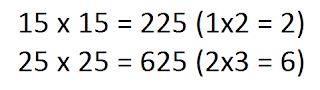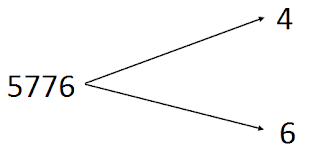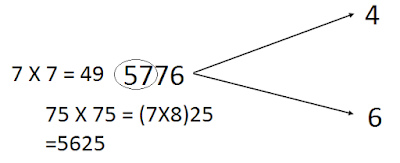Finding Square Roots Easily (Vedic Math)

Hello, Guys!!! I’m back with another post on Vedic Math that is going to keep you glued rooted to your spot 😆. This time I’m going to teach you how to calculate the square roots of perfect squares faster…

With a little practice, this method can let you calculate the roots in a matter of 5 seconds, whereas if you had used the traditional method, you would still be stuck with your question 😏

Things you need to know:-

Perfect square which has unit digit 1 will have square root with the last digit as 1 or 9

Perfect square which has unit digit 4 will have square root with the last digit as 2 or 8

Perfect square which has unit digit 5 will have square root with the last digit as 5

Perfect square which has unit digit 6 will have square root with the last digit as 4 or 6

Perfect square which has unit digit 9 will have square root with the last digit as 3 or 7

Perfect square which has unit digit 0 will have square root with the last digit as 0

And most importantly… you need to know this handy little trick for the method to work.

If you need to find the square of a number which ends with 5 you just write 25 at the end and multiply the remaining digits with its successor. Here are a couple of examples to help you understand better…So, now that you know everything to make this trick work… here’s The Thing itselfHuh?! Someone called me???
I'm sorry for that interruption... So without further adieu,

Let us begin!!

For this post let’s try to find 5776

1) Firstly, consider the last 2 digits- 76, and find the last number of the square root referring above… in this case, 4 or 62) Next, consider the remaining digits, 57 and find a number whose square is nearest to 57 but not above it, 7 x 7 = 49, therefore 7 in this case.3) So this number can have square root as either 76 or 74, to know which is the correct answer read further

4) Now we take the number between 76 and 74 i.e. 75 and find its square using the method given above -

75 x 75 = (7x8)25

= 56255) If the answer obtained in step 4 is greater than the number we want to find the root of, we take the greater option (6), if it is less than the original number, we take the lesser option (4)

6) As 5625 < 5776, therefore 5776 = 76

Some words of caution:-

·        Only works on perfect squares.

·        If the number ends in 5 or 0, then you don’t need to follow steps 3 – 6
Tired of your slow and inaccurate multiplication? Want to multiply faster? Here's something that might help you
All your reviews are highly appreciated, and I hope that you find this trick useful. Share this with your friends (it helps me a lot) and stay tuned for more on highschoolpedia.com . Any doubt would be cleared in the comments section belowSee you later

Have a nice day!!! ☺